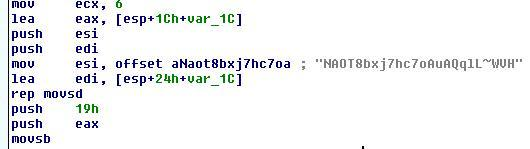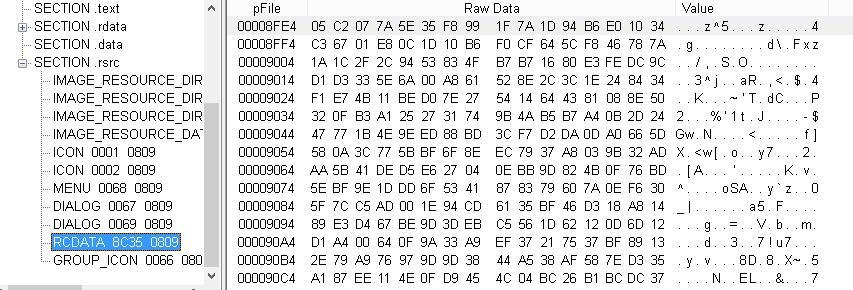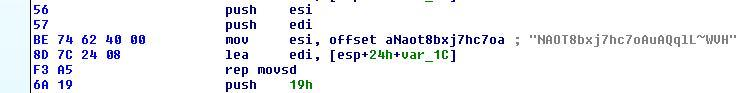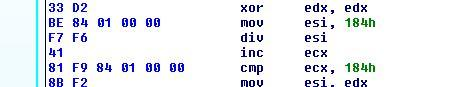# De-crypting a TrickBot Crypter

Introduction

TrickBot has utilized their own crypting service for some time now and it has been frequently updated over time. The latest version utilizes RC4 with a twist and is also a perfect example for writing a simple unpacker while at the same time being forced to analyze a slightly modified encryption routine.

Static Analysis

The crypter is not very obfuscated which makes static analysis fairly trivial, but there are a few potential pitfalls that an aspiring reverse engineer can have problems with. The first think it does is copy a hardcoded string:There is a big takeaway here the string is 0x18 bytes long if you are counting it as a standard C string as you would not count the terminating NULL byte, however here the hardcoded value used to copy the string is 0x19.

``````>>> a = 'NAOT8bxj7hc7oAuAQqlL~WVH'
>>> len(a)
24
>>> 0x19
25 ``````

If this is the key then some RC4 implementations and analysts could mistakenly try to use the string without the null terminator, it’s important to always remember that you are dealing with bytes at the lowest level and such a simple thing as not accounting for a NULL byte could end up tripping you up. For now we will remember this piece of information as we move forward.

The next thing done is LoadLibrary is used to check for the existence of ‘mshta.exe’, then a hardcoded string is converted to it’s integer value using ‘atoi’ and then passed to a function.

Looking at the only sub function within this function, we see it called in a loop. We find a function performing a common hashing technique found in malware. This is a ror-13 hash which is being used in a technique that uses hash values to resolve function dependencies. This is so analysts can’t easily tell functionality in a disassembler or through a strings utility.

The function will capitalize all the characters first though, so we need to account for that if we want to recreate it:

``````>>> a = 'virtualallocexnuma'.upper()
>>> a 'VIRTUALALLOCEXNUMA'
>>> h = 0
>>> for c in a:
...   h = ror(h, 13)
...   h += ord(c)
...
>>> h
383669855``````

This crypter is actually well known for it’s usage of VirtualAllocExNuma for allocating the memory section it will use for the next layer but where is this next layer? A look at the next block of code shows that the crypter stub is now retrieving a specific resource from itself.

Taking a look at this resource shows that it does appear to be some kind of obfuscated or encoded data:This data is copied over after allocating a section of memory using VirtualAllo-cExNuma which was previously resolved, and then the address of this memory along with the address of the hardcoded string from before is passed oﬀ to another function. After that function call it appears the memory address is then executed, so this data will be decoded by this function and will then be executable code!

Taking a look into this function shows a routine that might look familiar to any of you that have previously look at RC4 encryption routines that have been disassembled but something odd is going on normally this first SBOX initialization loop is 256 bytes but here we have 0x184.

After the SBOX initialization begins the KSA(Key-scheduling algorithm) portion of RC4 but once again we are left with the routine now using 0x184. Normal RC4 SBOx initialization for KSA is below:

``````S = list(range(256))
j = 0
out = []
for i in range(256):
j = (j + S[i] + ord( key[i % len(key)] )) % 256
S[i] , S[j] = S[j] , S[i] ``````

So in the instance of an extended box we need to account for that by changing a few things, first we need to directly extend S:

``S = list(range(0x184))``

We also need to keep the values with a normal byte range of 0x0-0xﬀ

``S = [x&0xff for x in S]``

The for loop will need to iterate over the entire extended SBOX so it will also need to be adjusted:

``for i in range(0x184): ``

Also our ‘j’ value for performing our swap, the previous ‘% 256’ was used to make sure ‘j’ did not go out of bounds of the SBOX so this value also needs to be adjusted:

``j = (j + S[i] + ord( key[i % len(key)] )) % 0x184``

After KSA in RC4 comes PRGA(Pseudo-random generation algorithm) which is the process by which the keystream data is generated in RC4, normally you will see this routine and XORing the data combined in order to save space:

So once again we have 0x184 used accounting for the newly extended SBOX, PRGA in normal RC4 while directly XORing the data looks like this:

``````i = j = 0
for char in data:
i = ( i + 1 ) % 256
j = ( j + S[i] ) % 256
S[i] , S[j] = S[j] , S[i]
out.append(chr(ord(char) ˆ S[(S[i] + S[j]) % 256]))``````

The ‘i’ and ‘j’ values are once again index values into performing a swap within the SBOX so they will need to be extended:

``````i = j = 0
for char in data:
i = ( i + 1 ) % 0x184
j = ( j + S[i] ) % 0x184``````

Also the last line can seem a bit confusing at first but the ‘% 256’ is once again used to prevent an out bounds on the SBOX after adding together the values at ‘i’ and ‘j’ indexes within the SBOX. The wikipedia article for RC4 is probably a bit more clear on this than me:

``exchanges the values of S[i] and S[j] then uses the sum S[i] + S[j] (modulo 256) as an index to fetch a third element of S (the keystream value K below) ``

as an index to fetch a third element of S (the keystream value K below)

So for our purposes we need to extend the bound check to 0x184:

``````    S[i] , S[j] = S[j] , S[i]
out.append(chr(ord(char) ˆ S[(S[i] + S[j]) % 0x184])) ``````

Our full script for testing:

``````import sys
def rc4_crypt(data, key):
S = list(range(0x184))
S = [x&0xff for x in S]
j = 0
out = []
for i in range(0x184):
j = (j + S[i] + ord( key[i % len(key)] )) % 0x184
S[i] , S[j] = S[j] , S[i]
i = j = 0
for char in data:
i = ( i + 1 ) % 0x184
j = ( j + S[i] ) % 0x184
S[i] , S[j] = S[j] , S[i]
out.append(chr(ord(char) ˆ S[(S[i] + S[j]) % 0x184]))
return ''.join(out)

def dump_file(key, src, dst):

src_file = open(src, 'rb')
src_file.close()
decrypted_pe = rc4_crypt(file_content, key)
dst_file = open(dst, 'wb')
dst_file.write(decrypted_pe)
dst_file.close()

key = "NAOT8bxj7hc7oAuAQqlL~WVH"+'\x00'
t = rc4_crypt(data,key) ``````

The output does appear to be executable code:

``````>>> t[:100]
'\xe8\x00\x00\x00\x00X\x89\xc3\x05:\x05\x00\x00\x81\xc3:\x9d\x02\x00h\x01\x00\x00 \x00h\x05\x00\x00\x00ShEwb0P\xe8\x04\x00\x00\x00\x83\xc4\x14\xc3\x83\xecH\x83d\$\x18 \x00\xb9Lw&\x07SUVW3\xf6\xe8"\x04\x00\x00\xb9I\xf7\x02x\x89D\$\x1c\xe8\x14\x04\x00 \x00\xb9X\xa4S\xe5\x89D\$ \xe8\x06\x04\x00\x00\xb9\x10\xe1'``````

There is also a PE file inside:

``````>>> t.find('This program')
1421
>>> t[1300:1500]
'k\xff\xff\xff3\xc0_ˆ][\x83\xc4\x10\xc3\x8bt\$\x10\x8bD\x16\$\x8d\x04X\x0f\xb7\x0c \x10\x8bD\x16\x1c\x8d\x04\x88\x8b\x04\x10\x03\xc2\xeb\xdbMZ\x90\x00\x03\x00\x00 \x00\x04\x00\x00\x00\xff\xff\x00\x00\xb8\x00\x00\x00\x00\x00\x00\x00@\x00\x00\x00 \x00\x00\x00\x00\x00\x00\x00\x00\x00\x00\x00\x00\x00\x00\x00\x00\x00\x00\x00\x00 \x00\x00\x00\x00\x00\x00\x00\x00\x00\x00\x00\x00\xc0\x00\x00\x00\x0e\x1f\xba\x0e \x00\xb4\t\xcd!\xb8\x01L\xcd!This program cannot be run in DOS mode.\r\r\n\$\x00 \x00\x00\x00\x00\x00\x00\xe5\xb5\xe8\xc9\xa1\xd4\x86\x9a\xa1\xd4\x86\x9a\xa1\xd4 \x86\x9a\xfa\xbc\x87\x9b\xa2\xd4\x86\x9a\xa1\xd4\x87\x9a\xa3'``````

The functionality of the crypter so far then is as follows:

• Decode resource section
• Detonate the newly decoded data which has the unpacked payload inside of it

Unpacker

To create an unpacker we need to be able to retrieve:

• RC4 Key
• Size of SBOX
• Resource section

For finding the values in the binary we are going to use python-yara, this lets us utilize YARA for both detection and also finding our way around the stub of the packer.

For getting the RC4 key we have a few options but I decided to utilize YARA so we can work through a few problems you might run into when going this route. If you remember the key is copied over:Many times you can find things within the stub of a crypter such as this which will remain very similar or almost even static in their construction, so signaturing on this copy sequence with an oﬀset makes me think this structure will remain somewhat consistent.

``\$snippet1 = {be ?? ?? ?? 00 8d 7c 24 [1-2] f3 a5}``

For the SBOX Size we can do something similar:``\$sbox_size = {be ?? ?? 00 00 f7 f6 [0-1] 81} ``

This makes our embedded yara rule for our script:

``````rule_source = '''
rule TrickBot {
meta:
author = "jreaves"
description = "TrickBot Crypter 2019/2020"
strings:
\$snippet1 = {be ?? ?? ?? ?0 8d 7c 24 [1-2] f3 a5}

\$sbox_size = {be ?? ?? 00 00 f7 f6 [0-1] 81}
condition:
(\$snippet1 and \$sbox_size)
} '''``````

For utilizing it I use a modified version of a function that Graham Austin wrote for a CAPE sandbox decoder.

``````#From Graham Austin
def yara_scan(raw_data, rule_name):
yara_rules = yara.compile(source=rule_source)
matches = yara_rules.match(data=raw_data)
for match in matches:
if match.rule == 'TrickBot':
for item in match.strings:
if item == rule_name:

This function simply scans data and then returns a list of hits, to utilize the data we have so far we will also need a more generic RC4 function that takes the SBOX size value along with the key and data:

``````def decode_data(data, key, sz):
S = list(range(sz))
S = [x&0xff for x in S]
j = 0
out = []
for i in range(sz):
j = (j + S[i] + ord( key[i % len(key)] )) % sz
S[i] , S[j] = S[j] , S[i]
i = j = 0
for char in data:
i = ( i + 1 ) % sz
j = ( j + S[i] ) % sz
S[i] , S[j] = S[j] , S[i]
out.append(chr(ord(char) ˆ S[(S[i] + S[j]) % sz]))
return ''.join(out) ``````

We also need a function for retrieving the resource sections from a PE file:

``````def get_rsrc(pe):
ret = []
for resource_type in pe.DIRECTORY_ENTRY_RESOURCE.entries:
if resource_type.name is not None:
name = str(resource_type.name)
else:
name = str(pefile.RESOURCE_TYPE.get \ (resource_type.struct.Id))
if name == None:
name = str(resource_type.struct.name)
if hasattr(resource_type, 'directory'):
for resource_id in resource_type.directory.entries:
if hasattr(resource_id, 'directory'):
for resource_lang in resource_id.directory.entries:
data = pe.get_data( \ resource_lang.data.struct. \ OffsetToData,resource_lang. \ data.struct.Size)
ret.append((name,data, \ resource_lang.data. \ struct.Size,resource_type))
return ret``````

Now we can leverage our scanning function from earlier to get our RC4 key and SBOX size value, but the key value is actually an oﬀset so we will need to adjust this value to actually find the key string. There’s a few ways to go about this but I will normally just subtract the PE ImageBase value from the recovered memory address and then use python PEFILE librarys memory mapping.

``````if __name__ == "__main__":
conf = {}
pe = pefile.PE(data=data)
mapped = pe.get_memory_mapped_image()
snippet = yara_scan(data, '\$snippet1')
if not snippet:
print("Key fail find")
for snipp in snippet:
offset = int(snipp)
if len(key) < 40:
break ``````

There is other ways to do this but this is a good way to tell you that if you aren’t familiar with whatever file format you are looking at then you should become familiar with it when trying to script out things like this. I also added a quick check at the end which is more like a sanity check.

Next we scan for the SBOX size value:

``````snippet = yara_scan(data, '\$snippet1')
if not snippet:
print("Key fail find")
for snipp in snippet:
offset = int(snipp)
if len(key) < 30:
break ``````

Then simply enumerate every resource section looking for RCDATA resources:

``````r = get_rsrc(pe)
i = 0
for rsrc in r:
if 'RCDATA' in rsrc:
temp = decode_data(rsrc, key+'\x00', sz)
open(sys.argv+'_'+str(i)+'.dec', 'wb').write(temp)
o = temp.find('MZ')
print("Writing PE file")
open(sys.argv+'_'+str(i)+'.dec.bin', 'wb').write(temp[o:])
i += 1``````

There are definately ways to improve this process but I decided to keep it as simple as possible in the hopes that it would be easier to learn. A good way to begin trying to improve the script would be to find more samples that you can study and use this data to expand the capabilities of the script for more variants of the crypter.

Terminology

Stub – This is a program that expects data to exist within itself that isn’t actually there. The data gets filled in by the builder for a crypter/packer and then the stub functions normally and unpacks the data.

References

1: RC4